## Right Triangle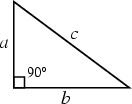A Triangle with an Angle of 90° (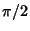radians). The sides,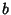, and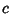of such a Triangle satisfy the Pythagorean Theorem. The largest side is conventionally denotedand is called the Hypotenuse.

For any three similar shapes on the sides of a right triangle,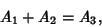(1)

which is equivalent to the Pythagorean Theorem. For a right triangle with sides,, and Hypotenuse, let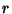be the Inradius. Then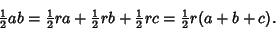(2)

Solving forgives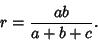(3)

But any Pythagorean Triple can be written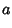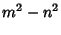(4)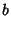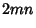(5)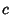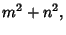(6)

so (5) becomes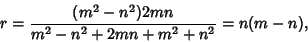(7)

which is an Integer whenandare integers.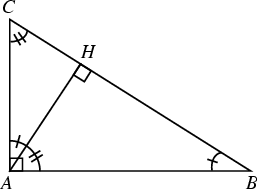Given a right triangle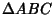, draw the Altitude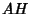from the Right Angle. Then the triangles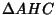and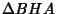are similar.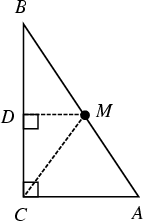In a right triangle, the Midpoint of the Hypotenuse is equidistant from the three Vertices (Dunham 1990). This can be proved as follows. Given, let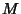be the Midpoint of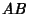(so that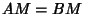). Draw, then since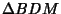is similar to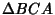, it follows that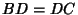. Since bothand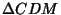are right triangles and the corresponding legs are equal, the Hypotenuses are also equal, so we have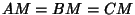and the theorem is proved.

See also Acute Triangle, Archimedes' Midpoint Theorem, Brocard Midpoint, Circle-Point Midpoint Theorem, Fermat's Right Triangle Theorem, Isosceles Triangle, Malfatti's Right Triangle Problem, Obtuse Triangle, Pythagorean Triple, Quadrilateral, RAT-Free Set, Triangle

References

Beyer, W. H. (Ed.) CRC Standard Mathematical Tables, 28th ed. Boca Raton, FL: CRC Press, p. 121, 1987.

Dunham, W. Journey Through Genius: The Great Theorems of Mathematics. New York: Wiley, pp. 120-121, 1990.